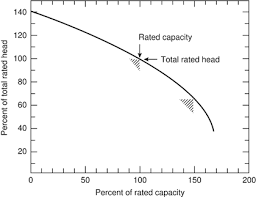## How to Calculate and Solve for Capacity of the Sprinkler System | Irrigation Water RequirementThe image above represents capacity of the sprinkler system.

To compute for the capacity of the sprinkler system, four essential parameters are needed and these parameters are Area to be Irrigated (A), Net Depth of Water Application (d), Number of Drop allowed for the Completion of One Irrigation (F), Number of Actual Operating Hours per Day (H) and Water Application Efficiency (E).

The formula for calculating capacity of the sprinkler system:

Q = 27800 . A . d / F . H . E

Where:

Q = Capacity of the Sprinkler System
A = Area to be Irrigated
d = Net Depth of Water Application
F = Number of Drop allowed for the Completion of One Irrigation
H = Number of Actual Operating Hours per Day
E = Water Application Efficiency

Let’s solve an example;
Find the capacity of the sprinkler system when the area to be irrigated is 2, the net depth of water application is 8, the number of drop allowed for the completion of one irrigation is 10, the number of actual operating hours per day is 6 and the water application efficiency is 4.

This implies that;

A = Area to be Irrigated = 2
d = Net Depth of Water Application = 8
F = Number of Drop allowed for the Completion of One Irrigation = 10
H = Number of Actual Operating Hours per Day = 6
E = Water Application Efficiency = 4

Q = 27800 . A . d / F . H . E
Q = 27800 . (2) . (8) / (10) . (6) . (4)
Q = 444800 / 240
Q = 1853.3

Therefore, the capacity of the sprinkler system is 1853.3.

Calculating for the Area to be Irrigated when the Capacity of the Sprinkler System, the Net Depth of Water Application, the Number of drop Allowed for the Completion of One Irrgiation, the Number of Actual Operating Hours per Day and the Water Application Efficiency is Given.

A = Q x F . H . E / 27800 . d

Where;

A = Area to be Irrigated
Q = Capacity of the Sprinkler System
d = Net Depth of Water Application
F = Number of Drop allowed for the Completion of One Irrigation
H = Number of Actual Operating Hours per Day
E = Water Application Efficiency

Let’s Solve an example;
Find the area to be irrigated when the capacity of the sprinkler system is 4, the net depth of water application is 6, number of drop allowed for the competion of one irrigation is 2, number of actual operating hours per day is 3 and the water application efficiency is 8.

This implies that;

Q = Capacity of the Sprinkler System = 4
d = Net Depth of Water Application = 6
F = Number of Drop allowed for the Completion of One Irrigation = 2
H = Number of Actual Operating Hours per Day = 3
E = Water Application Efficiency = 8

A = Q x F . H . E / 27800 . d
A = 4 x 2 . 3 . 8 / 27800 . 6
A = 192 / 166800
A = 0.00115

Therefore, the area to be irrigated is 0.00115.